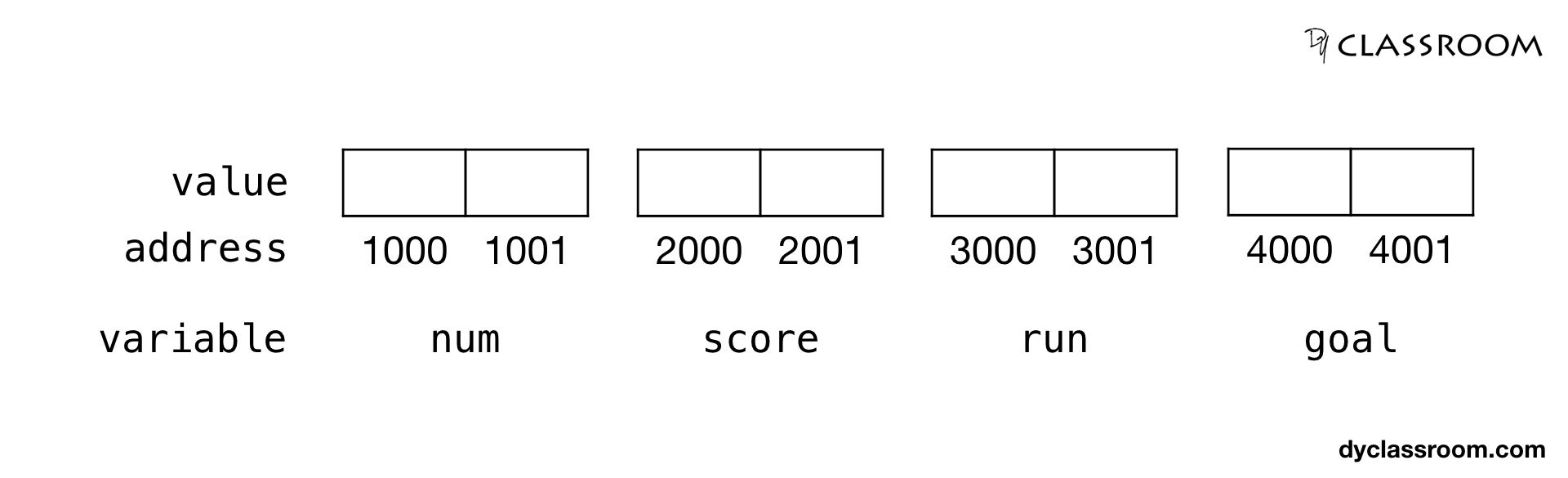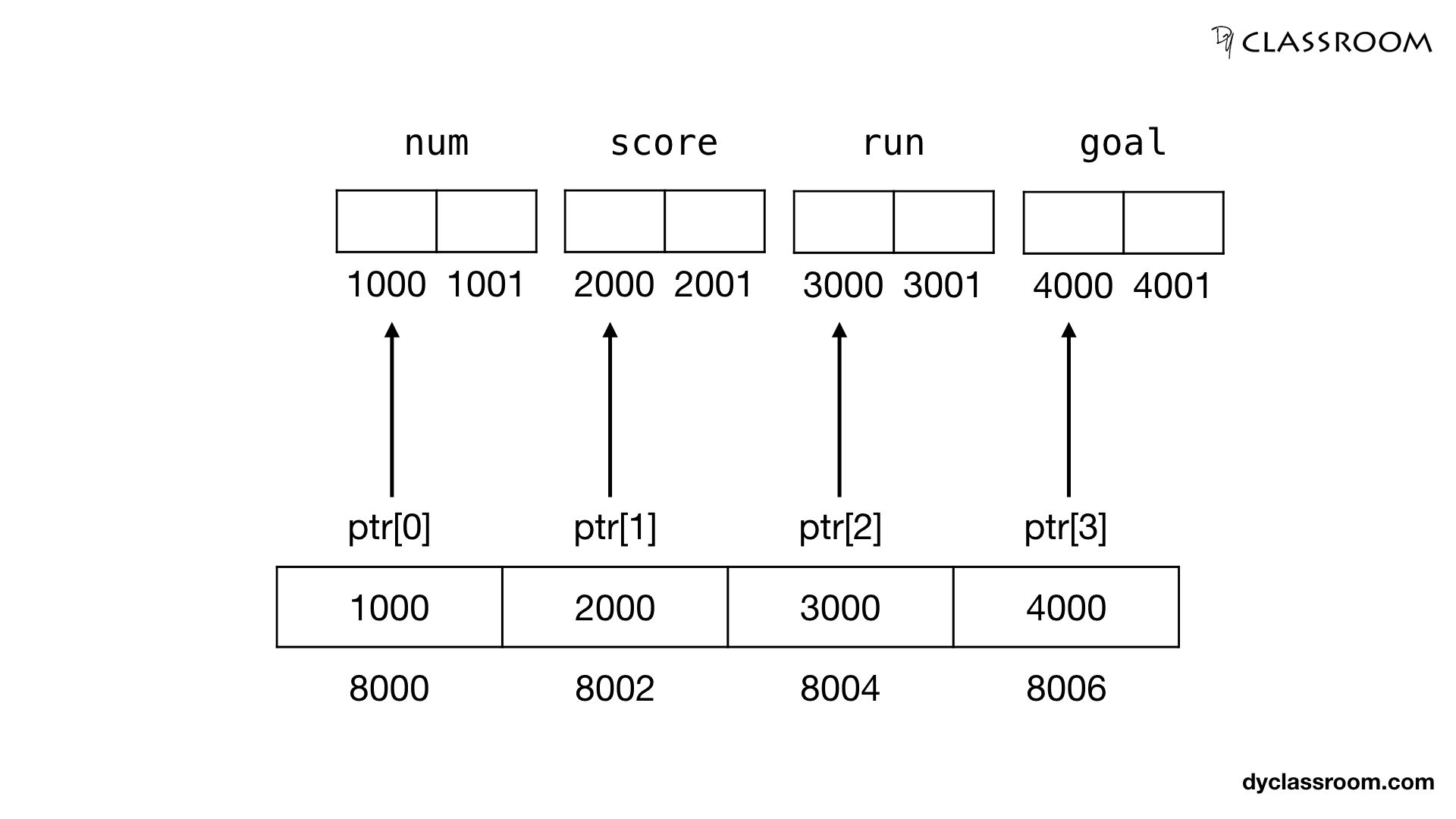# C - Array of Pointers

C ProgrammingIn this tutorial we will learn about array of pointers in C programming language.

We have so for learned about pointers and one dimensional arrays and pointers and two dimensional arrays. Feel free to checkout those tutorial.

## Creating variables

For this tutorial we will create four integer variables.

``````// integer variables
int num = 10;
int score = 12;
int run = 123;
int goal = 3;
``````

We can represent this in memory as follows.We are assuming that an integer value takes 2 bytes so, each variable is taking 2 bytes space in memory.

Those memory spaces are filled with integer value which is not shown in the picture above.

## Array of pointers

Since we have four integer pointers so, we can either create four separate integer pointer variables like `ptr1`, `ptr2`, `ptr3` and `ptr4`.

Or, we can create one single integer array of pointers `ptr` variable that will point at the four variables.

In the following example we are creating an array of integer pointers ptr of size 4.

``````// array of integer pointers
int *ptr;
``````

## Assign address to array of pointers

This step is similar to any other pointer variable. We get the address of a variable using the address of `&` operator and then save that address in the array of pointers.

In the following example we are saving the address of the integer variables `num`, `score`, `run` and `goal` in the integer array of pointers variable `ptr`.

``````// assign address of variables to ptr
ptr = &num;
ptr = &score;
ptr = &run;
ptr = &goal;
``````Assuming that an integer address value takes 2 bytes so, each pointer element size is 2 bytes. So, the array of integer pointer `ptr` takes memory space from 8000 to 8007 i.e., total 8 bytes (2 bytes for each element).

The first element of the array of integer pointer `ptr` holds the address of the `num` variable.

Similarly, the second element of the array of integer pointer `ptr` holds the address of the `score` variable.

The third element of `ptr` holds the address of the `run` variable and the fourth element of `ptr` holds the address of the `goal` variable.

## Access value of the variables via array of pointers

To access the value of the variables via array of pointers we have to use the value at the address of `*` operator.

``````// print value
printf("num: %d\n", *ptr);
printf("score: %d\n", *ptr);
printf("run: %d\n", *ptr);
printf("goal: %d\n", *ptr);
``````

### Complete code

``````#include <stdio.h>

int main(void) {

// integer variables
int num = 10;
int score = 12;
int run = 123;
int goal = 3;

// array of integer pointers
int *ptr;

// assign address of variables to ptr
ptr = #
ptr = &score;
ptr = &run;
ptr = &goal;

// print value
printf("num: %d\n", *ptr);
printf("score: %d\n", *ptr);
printf("run: %d\n", *ptr);
printf("goal: %d\n", *ptr);

return 0;
}
``````

Output:

``````num: 10
score: 12
run: 123
goal: 3
``````